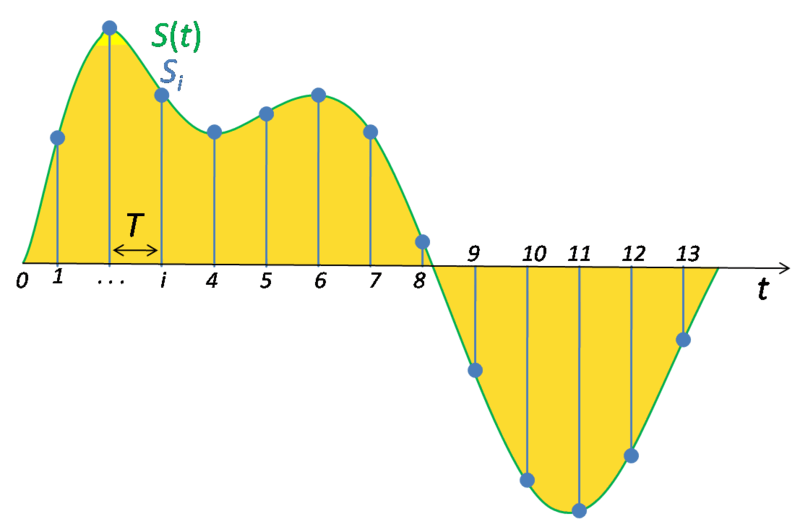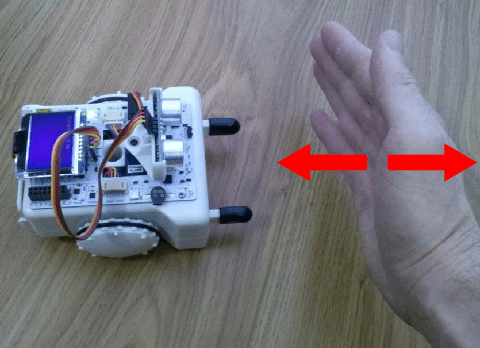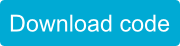# Signal Filtering

SparkiDuino
Medium

## Introduction

As you may have noted from previous lessons, sometimes the sensor reading function returns strange values. For example, an ultrasonic range finder reads (erroneous) really large distances from time to time, even when there is a near object in front of it. In this lesson we will learn some simple techniques that will help us to reduce the effects of the noise and false readings from the robot’s sensors. Also, we will introduce here some basic concepts about the analog to digital signal conversions used in Sparki.

## Analog to Digital Signal Conversions

Before start to work with Sparki’s sensors, let’s see some basic concepts about the way that our small robot receives information from the external world. As you may know, Sparki’s brain is a small digital microcontroller (MCU), specifically an Atmel ATMega32U4. And by digital we mean that in its inside, it deals with digital signals composed of only ones and zeros (where a digital one is represented by 5 Volts and an digital zero is represented by 0 Volts). Thus, in order to work with analog signals, which in our context are basically signals that can vary from 0 to 5 V in a continuous way, Sparki’s MCU has to translate them into ones and zeros. There are several techniques to do this conversion and Sparki’s MCU includes from factory an Analog to Digital Coverter (ADC). Let’s see first how it works in a generic way, so after having a basic understanding, we can deal with the specific details of our ADC. Please take a look to the following figure:Original image from Wikimedia Commons.

There, the green line represents the continuous analog signal, while the yellow surface is the area under the curve. As our small MCU can’t do much with that curve, the ADC enters in scene, taking samples of only a subset of the signal’s points (or values), and expressing each of these values with a binary number (an integer). As you may have guessed, the  blue points in the previous figure are representing the sampled points. So here, a few questions appear. For example: how many samples should the ADC take in order to represent the original signal? That’s a good question, so let’s talk about it a bit more.

Another important question about the conversion of a signal to the digital domain is the size of the numbers used to store each sampled point. For example, if we use just 8 bits to store a sampled value, we can represent it with only 256 different numbers. So, any point between the possible representations will be rounded to an approximate value. In the following figure, for example, we are showing an ADC technique (with 3 bits of resolution) where each sampled value (blue points) from the original signal (red curve) is mapped to a binary number below the sampled point, resulting in the blue signal. We can see clearly that the approximation is not really good with just 3 bits in this case:The quantity of bits used to store the sampled values is called the resolution of the ADC. As with the sample rate, a higher resolution means in general a better representation of the signal, but it also takes more memory space when storing it. And will need more processing power if we want to work mathematically with that signal. Sparki’s ADC features a 10 bits (maximum) resolution. This means that the analogRead function used inside the libraries for sensors like the Infrared Reflectance Sensors, or the Light Sensors can return numbers between 0 and 1023 (since 2^10 = 1024). Again, that’s good enough, as you may have seen in the previous lessons when working with Sparki’s sensors!

## Averaging Ultrasonic Range Finder Readings

Now that we have learned a few things about signal conversions, let’s start to work with real signals from the Sparki sensors. If you try the following code snippet, adapted from the Ultrasonic Ranger Finder page code, you will note that if you move your hand oscillating quickly towards and from the robot, you may see some sensor readings which are clearly false readings (with numbers like 150 cm, for example):Some of these readings could even be true, but we are not interested in them, since they may be the result of a temporary event that should not affect our robot’s behavior (for example something passed fast in front of the robot, thus not being a real obstacle if the robot is moving forward). So, to improve the confidence in our sensor readings, and if the speed of these readings is not critical, we can always apply a simple technique: the average. This way, instead of just printing the result of one single reading, we will take the value of 3 of them (this number is arbitrary, of course, and should be adjusted to our specific needs) and calculate the average (or arithmetic mean). This is the sum of all the read values, divided by the quantity of readings (or signal samples). Take a look to the following code:## A Generic Average Function

But what if we want to apply the average function to other sensors? Sparki has plenty of sensors, and calculating the arithmetic mean of their readings could be useful not just for the ultrasonic distance ranger, but for all of them. Even digital readings could be averaged (try it!). So, why not write a generic average function that we can use with any of these useful sensors? Let’s take a look to the following code: